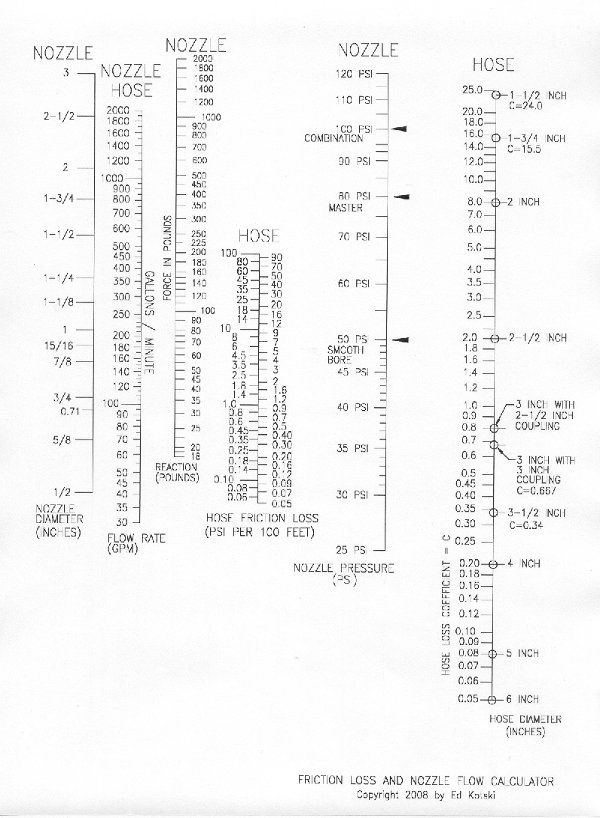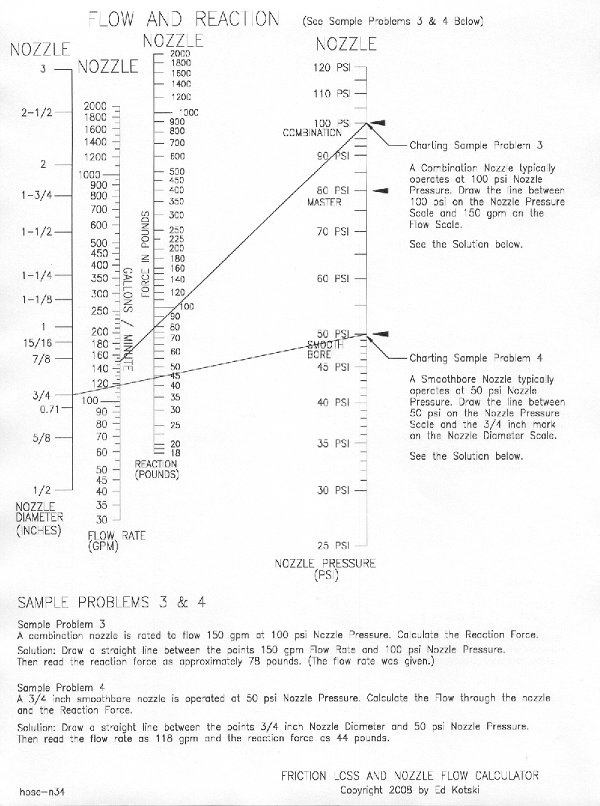Friction Loss Calculator and Pump Op Tool | JSBachFOA.Org

# Calculating Friction Loss, Nozzle Reaction, and Nozzle Flow Using the Hose and Nozzle Easy Chart

If you can draw a straight line, you can calculate friction loss, nozzle flow, and nozzle reaction forces in fire service hoselines. The chart, technically called a nomograph, acts as a vernier scale to quickly connect known information to find friction loss, nozzle reaction forces, and nozzle flow on the fire ground. To start, download and print the PDF Document.## Calculating Friction LossTo calculate hose friction loss per 100 ft of hose:

1. Locate the hose diameter on the Hose Diameter Scale
2. Locate the flow rate on the Flow Rate Scale
3. Connect these two points
4. Find the point where the line you drew crosses the Hose Friction Loss Scale. This is the friction loss in psi per 100 ft hose.

## Calculating Nozzle Flow and Reaction Forces### Combination Nozzle Calculations

1. Locate the operating pressure of the nozzle on the Nozzle Pressure Scale. For Combination Nozzles, this is typically 100 psi.
2. Locate the flow rate on the Flow Rate Scale.
3. Connect these two locations with a straight line
4. Locate the Nozzle Reaction Force where the line you drew crosses the Reaction Scale

### Smooth Bore Nozzle Calculations

1. Locate the nozzle diameter on the Nozzle Diameter Scale
2. Locate the nozzle operating pressure on the Nozzle Pressure Scale
3. Connect these two locations with a straight line
4. Locate the flow rate where the line you drew crosses the Flow Rate Scale

## Combining Friction Loss, Nozzle Flow, and Nozzle Reaction ForcesCombining all calculations on a single chart is done in two steps
1. Using the Nozzle Scales, connect the operating pressure and the nozzle diameter (smoothbore) or flow rate (combination nozzle)
2. Read the Reaction Force where this line crosses the Reaction Force Scale and the Flow Rate from the Flow Rate Scale. DO NOT read the friction loss from this line.
3. Connect the hose diameter to the flow rate and read the friction loss per 100 ft of hose where the new line crosses the Hose Friction Loss Scale